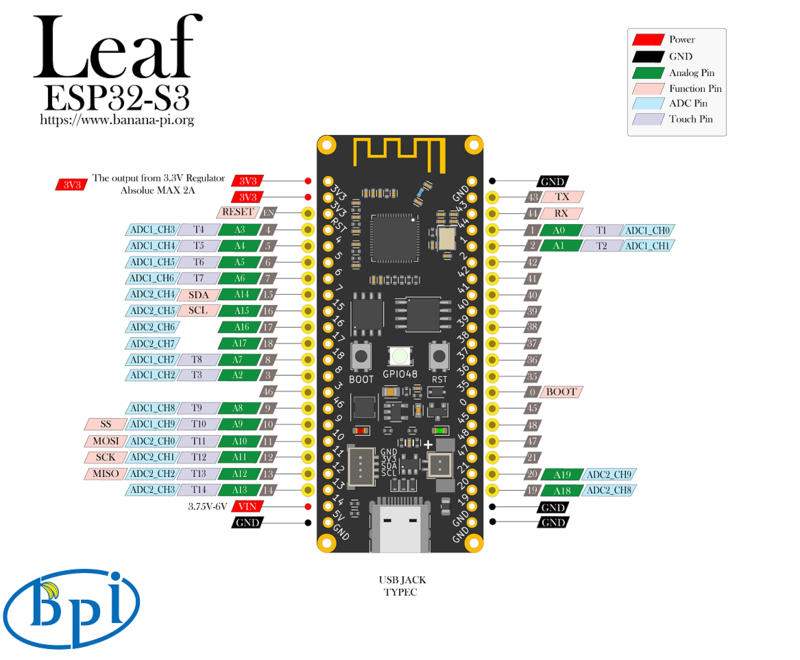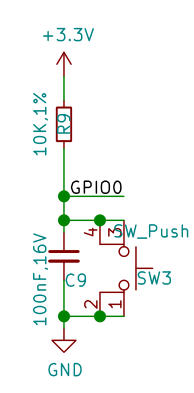# Banana Pi BPI-Leaf-S3 with ESP32-S3, use key interrupts to control neopixelBpi-leaf-s3 has two keys, BOOT and RST, RST control chip hardware reset, and BOOT is connected to GPIO0, its circuit is shown in the following figure.It can be seen that when the development board is powered on normally, when the BOOT button is not pressed by GPIO0, a resistor is connected to 3.3V in series, which is a high potential. When the BOOT button is pressed, GPIO0 will be directly grounded, which indicates a low potential. The ESP32-S3 chip determines whether the button is pressed by detecting the potential of the GPIO pin.

## MicroPython

Micropython Establishment of operating environment - Banana Pi Wiki (banana-pi.org)

MicroPython GPIO * interrupt program machine.Pin.irq documents

In the program, by detecting the trigger mode of GPIO interrupt, a set of interrupt program can be designed to record the number of times the key is pressed, and the color of the color lamp can be controlled by judging the number of times the key has been pressed.

``````from machine import Pin
from neopixel import NeoPixel
from array import array
import time
import micropython

micropython.alloc_emergency_exception_buf(100)

p_48 = Pin(48, Pin.OUT)
np = NeoPixel(p_48, 1,bpp=3, timing=1)

p0 = Pin(0,Pin.IN,Pin.PULL_UP)
trig_locks = array('B',)
trig_timeticks_list = array('L',[0,0])
count = array('L',)

def p0_irq(pin):
if pin.value()==0 and trig_locks==0:
trig_timeticks_list=time.ticks_ms()
trig_locks=1
elif pin.value()==1 and trig_locks==1:
trig_timeticks_list=time.ticks_diff(time.ticks_ms(),trig_timeticks_list)
trig_locks=0
if trig_timeticks_list >= 20:
count = count + 1
if count > 8:
count = 0

p0.irq(handler=p0_irq,trigger= Pin.IRQ_FALLING | Pin.IRQ_RISING )

RED = (255, 0, 0)
ORANGE = (255, 100, 0)
YELLOW = (255, 255, 0)
GREEN = (0, 255, 0)
CYAN = (0, 255, 255)
BLUE = (0, 0, 255)
PURPLE = (180, 0, 255)
WHITE = (255, 255, 255)
OFF = (0, 0, 0)

color_list = [RED,ORANGE,YELLOW,GREEN,CYAN,BLUE,PURPLE,WHITE,OFF]
brightness = 0.1

while True:
print (count)
i = color_list[count]
color = (round(i*brightness),round(i*brightness),round(i*brightness))
np = color
np.write()
time.sleep(0.1)
``````

demo video:

Banana Pi BPI-Leaf-S3 wiki page: https://wiki.banana-pi.org/BPI-Leaf-S3

Banana Pi BPI-Leaf-S3 with ESP32-S3 & microPython Neopixel show